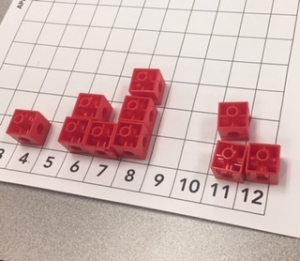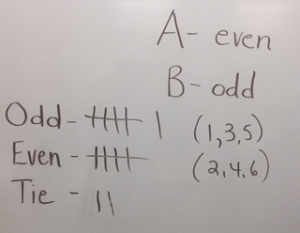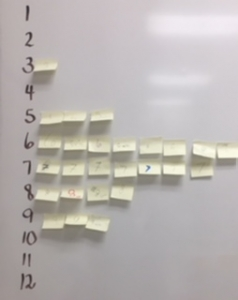# Probability – Lucky Numbers

Recently, I planned and implemented this probability lesson in both a Grade 5 and a Grade 3 classroom respectively.  This is a example of a lesson that could be used in any Primary/Junior classroom and would also be an easy game to send home for students to play with family members.  Many substitutions could be made for the snap cubes e.g., buttons, bingo chips, coins, bread tags

I started by saying that I keep hearing people say that some numbers are luckier than others and I want them to help me discover whether this is true or not.

Lucky Numbers

Big Idea:  We can estimate or make predictions about a probability situation by using an appropriate model and conducting an experiment.

Learning Goals:  We want students to understand:

• that they can make thoughtful predictions or estimations about this probability situation by looking at the numbers being used;
• that with probability situations things may not turn out as they expected, but that does not make their prediction based on that information wrong;
• that the more times we try the experiment and combine that information the close the information will get to their thoughtful prediction.

Curriculum Expectations:  By the end of …• Gr. 1:  students will describe the likelihood that everyday events will occur, using mathematical language (i.e., impossible, unlikely, less likely, more likely, certain);
• Gr. 2:  students will describe the probability that an event will occur through investigation with simple games and probability experiments;
• Gr. 3:  students will predict the frequency of an outcome in a simple probability experiment or game;
• Gr. 4:  students will determine through investigation, how many number of repetitions of a probability experiment can affect the conclusions;
• Gr. 5:  students represent using a common fraction, the probability that an event will occur in simple games and probability experiments.

Curriculum Connections:  basic number facts, decomposing numbers, even/odd, graphing

Three – Part Inquiry Lesson

Minds On:

Play:  Is This Game Fair?

Divide the class into pairs (Player A & Player B).   Player A rolls a number cube (regular dice).  If the number is even, Player A gets a point and if an odd number comes up Player B gets a point.  Take turns rolling and keeping score.  After everyone has completed one game (10 rolls in total) gather the data from the class. With a show of hands ask how many A’s won, B’s won and how many ties?  Record this using tallies.(See photo)  Ask:  Is this game fair?  Why or why not?

Action:

Task #1:  “Winning Number” – The object of this task is to find a “winning” number.  Each student has their own pair of number cubes and a game board.  After rolling the 2 number cubes, they add the 2 together and put a snap cube above the correct sum on the board e.g., 6 and 4 goes above 10.  The winning number is the one that gets to the top first.  Write your winning number on a post-it note and put it in the appropriate spot on the class graph (see photo).

Consolidation Questions:

1. Is it possible to get all numbers?2. Are some numbers “luckier” than others?
3. Are they really lucky?  Why or why not?

Task #2:  “Clear the Board” – This game is played in pairs and the winner is the one who clears their board first.

Each player has their own game board and 10 snap cubes.  Using the 10 snap cubes, place them on the board above the numbers wherever you want.  You can place more than one cube above a number.  The first player rolls 2 number cubes and adds them together.  If there is a cube above the sum, the player takes one cube off that number.  Whoever clears his/her board first wins the game.  Play it again.

Consolidation Questions:

1. How did you decide where to place your cubes when you started the game?
2. Did you change your mind after you played the game the first time?
3. Does every number have the same chance of being rolled?
4. Which numbers are the most likely to come up?  least likely?
5. Is it possible for a 2 to be rolled every time?  Is that likely?

Thinking back to our first game, “Even and Odd”, is this game still fair, if we use 2 number cubes?  How could we find out? (This could be next day’s investigation.)

Closing:  The object of this game is to “stay standing”.

• Ask students to choose a “secret” number between 2 and 12 and write it down.
• Everybody starts by standing up.
• Teacher rolls 2 number cubes and calls out the total.  If your number is called, “sit down”.
• After 10 rolls, the winners are the people still standing.